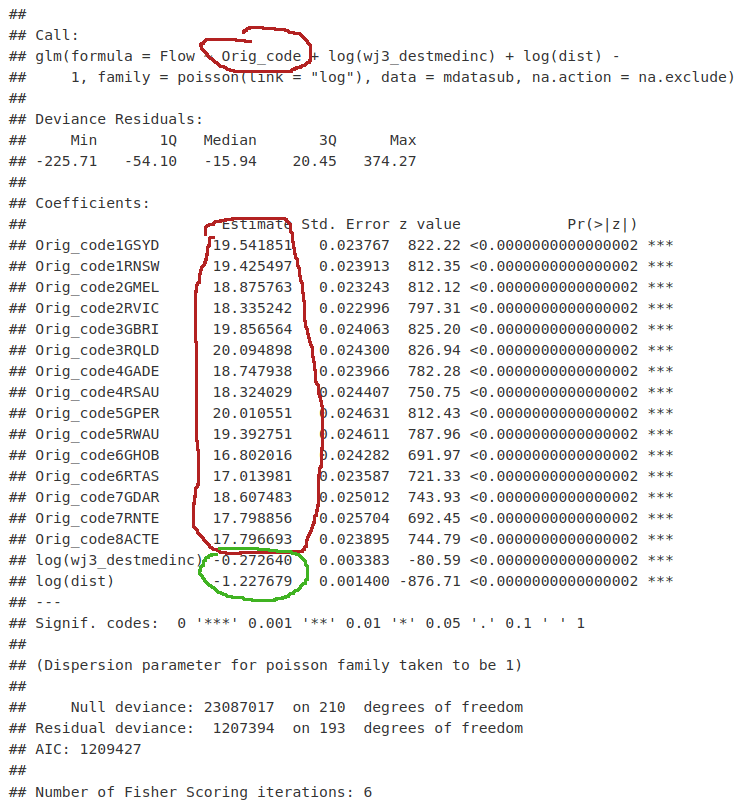# #StackBounty: #regression-coefficients #spatial #poisson-regression #spatial-interaction-model Struggling to make sense of coefficients…

### Bounty: 50

I’m having a hard time trying to understand what is the meaning of the coefficients of a production-constrained spatial interaction model re-specified as a Poisson linear regression model.

Following this guide to run a production-constrained spatial interaction model (section 2):

Which is the practical part of this paper:

https://www.australianpopulationstudies.org/index.php/aps/article/view/38

The model is re-specified as a Poisson regression model.

Here’s the output of the model in the guide.I suppose that those coefficients can’t be interpreted as in a linear regression, because the whole equation ins exponentiated.

Thanks in advance, any feedback will be highly appreciated.

Get this bounty!!!

This site uses Akismet to reduce spam. Learn how your comment data is processed.